3.1 Functions and function notation  (Page 9/21)

 Page 9 / 21

We will see these toolkit functions, combinations of toolkit functions, their graphs, and their transformations frequently throughout this book. It will be very helpful if we can recognize these toolkit functions and their features quickly by name, formula, graph, and basic table properties. The graphs and sample table values are included with each function shown in [link] .

Toolkit Functions
Name Function Graph
Constant $f\left(x\right)=c,\text{\hspace{0.17em}}$ where $c$ is a constant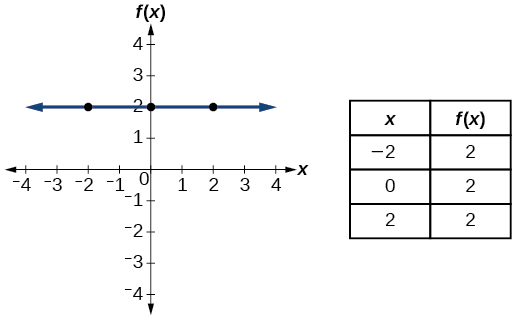Identity $f\left(x\right)=x$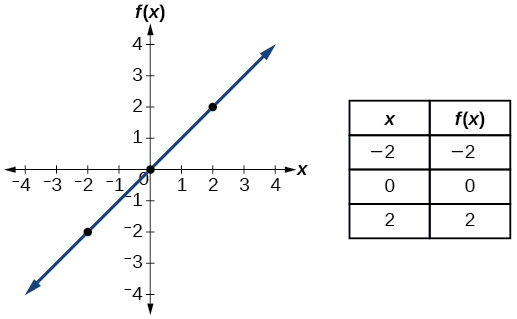Absolute value $f\left(x\right)=|x|$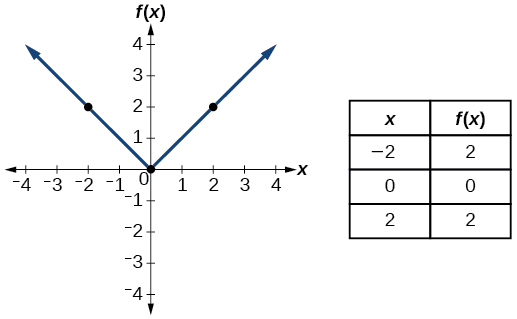Quadratic $f\left(x\right)={x}^{2}$Cubic $f\left(x\right)={x}^{3}$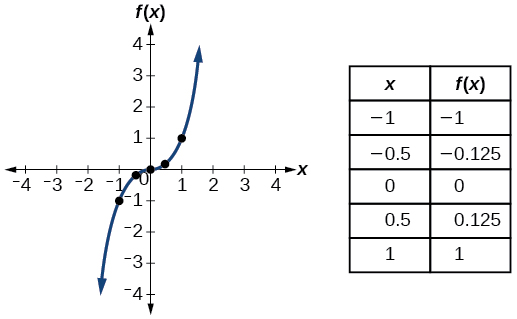Reciprocal $f\left(x\right)=\frac{1}{x}$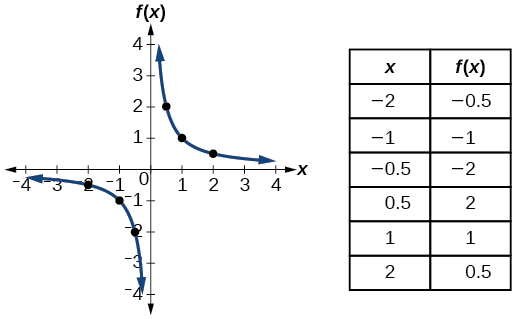Reciprocal squared $f\left(x\right)=\frac{1}{{x}^{2}}$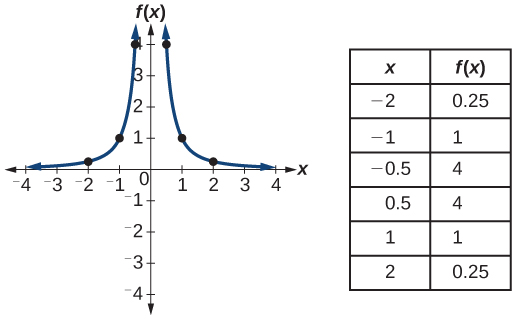Square root $f\left(x\right)=\sqrt{x}$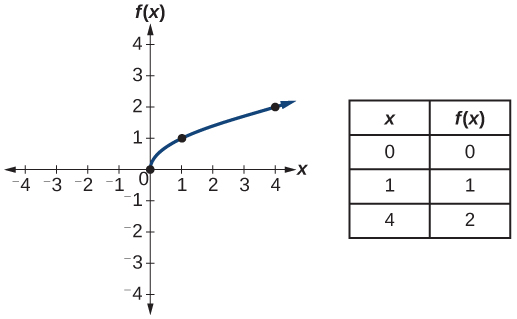Cube root $f\left(x\right)=\sqrt{x}$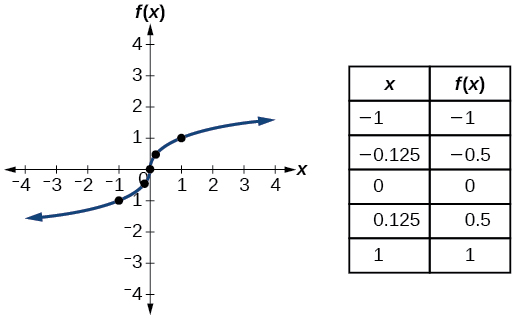Access the following online resources for additional instruction and practice with functions.

Key equations

 Constant function $f\left(x\right)=c,$ where $\text{\hspace{0.17em}}c\text{\hspace{0.17em}}$ is a constant Identity function $f\left(x\right)=x$ Absolute value function $f\left(x\right)=|x|$ Quadratic function $f\left(x\right)={x}^{2}$ Cubic function $f\left(x\right)={x}^{3}$ Reciprocal function $f\left(x\right)=\frac{1}{x}$ Reciprocal squared function $f\left(x\right)=\frac{1}{{x}^{2}}$ Square root function $f\left(x\right)=\sqrt{x}$ Cube root function $f\left(x\right)=\sqrt{x}$

Key concepts

• A relation is a set of ordered pairs. A function is a specific type of relation in which each domain value, or input, leads to exactly one range value, or output. See [link] and [link] .
• Function notation is a shorthand method for relating the input to the output in the form $\text{\hspace{0.17em}}y=f\left(x\right).\text{\hspace{0.17em}}$ See [link] and [link] .
• In tabular form, a function can be represented by rows or columns that relate to input and output values. See [link] .
• To evaluate a function, we determine an output value for a corresponding input value. Algebraic forms of a function can be evaluated by replacing the input variable with a given value. See [link] and [link] .
• To solve for a specific function value, we determine the input values that yield the specific output value. See [link] .
• An algebraic form of a function can be written from an equation. See [link] and [link] .
• Input and output values of a function can be identified from a table. See [link] .
• Relating input values to output values on a graph is another way to evaluate a function. See [link] .
• A function is one-to-one if each output value corresponds to only one input value. See [link] .
• A graph represents a function if any vertical line drawn on the graph intersects the graph at no more than one point. See [link] .
• The graph of a one-to-one function passes the horizontal line test. See [link] .

Verbal

What is the difference between a relation and a function?

A relation is a set of ordered pairs. A function is a special kind of relation in which no two ordered pairs have the same first coordinate.

What is the difference between the input and the output of a function?

Why does the vertical line test tell us whether the graph of a relation represents a function?

When a vertical line intersects the graph of a relation more than once, that indicates that for that input there is more than one output. At any particular input value, there can be only one output if the relation is to be a function.

How can you determine if a relation is a one-to-one function?

sin^4+sin^2=1, prove that tan^2-tan^4+1=0
what is the formula used for this question? "Jamal wants to save \$54,000 for a down payment on a home. How much will he need to invest in an account with 8.2% APR, compounding daily, in order to reach his goal in 5 years?"
i don't need help solving it I just need a memory jogger please.
Kuz
A = P(1 + r/n) ^rt
Dale
how to solve an expression when equal to zero
its a very simple
Kavita
gave your expression then i solve
Kavita
Hy guys, I have a problem when it comes on solving equations and expressions, can you help me 😭😭
Thuli
Tomorrow its an revision on factorising and Simplifying...
Thuli
ok sent the quiz
kurash
send
Kavita
Hi
Masum
What is the value of log-1
Masum
the value of log1=0
Kavita
Log(-1)
Masum
What is the value of i^i
Masum
log -1 is 1.36
kurash
No
Masum
no I m right
Kavita
No sister.
Masum
no I m right
Kavita
tan20°×tan30°×tan45°×tan50°×tan60°×tan70°
jaldi batao
Joju
Find the value of x between 0degree and 360 degree which satisfy the equation 3sinx =tanx
what is sine?
what is the standard form of 1
1×10^0
Akugry
Evalute exponential functions
30
Shani
The sides of a triangle are three consecutive natural number numbers and it's largest angle is twice the smallest one. determine the sides of a triangle
Will be with you shortly
Inkoom
3, 4, 5 principle from geo? sounds like a 90 and 2 45's to me that my answer
Neese
answer is 2, 3, 4
Gaurav
prove that [a+b, b+c, c+a]= 2[a b c]
can't prove
Akugry
i can prove [a+b+b+c+c+a]=2[a+b+c]
this is simple
Akugry
hi
Stormzy
x exposant 4 + 4 x exposant 3 + 8 exposant 2 + 4 x + 1 = 0
x exposent4+4x exposent3+8x exposent2+4x+1=0
HERVE
How can I solve for a domain and a codomains in a given function?
ranges
EDWIN
Thank you I mean range sir.
Oliver
proof for set theory
don't you know?
Inkoom
find to nearest one decimal place of centimeter the length of an arc of circle of radius length 12.5cm and subtending of centeral angle 1.6rad
factoring polynomialByByBy